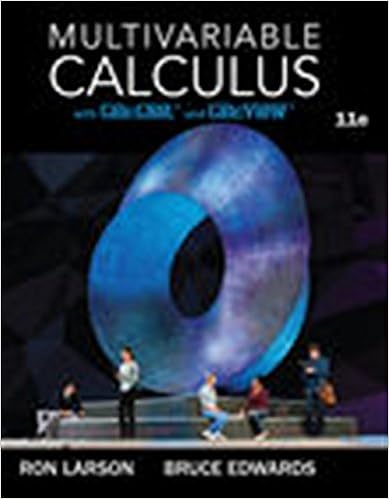# PHY31M1 Electrostatics.9 March 2017.pdf - 2 Electrostatics...

• Notes
• 19

This preview shows page 1 - 4 out of 19 pages.

##### We have textbook solutions for you!
The document you are viewing contains questions related to this textbook.The document you are viewing contains questions related to this textbook.
Chapter 12 / Exercise 69
Multivariable Calculus
LarsonExpert Verified
1 2. Electrostatics 2.1 Electric Field and Force The electric field Edat the field point ),,(Pzyxdue to the elemental source electric scalarcharge qdon a particle at point ),,(Pzyxis Fig. 2.1: Positions of field Pand source Ppoints and the displacement Rof Pfrom P204ˆddRqRE[11mVor CN] (2.1) where rrRrrRˆˆˆis the displacement of Pfrom P(see fig. 2.1), and 2121120mNCor mF10854.8(2.2) the free space permittivity in the SI unit of farad per metre (1mF). This Editself has the SI unit of newtons per coulomb (1CN) or volts per metre (1mV), while the electric scalar charge has the SI unit of coulomb (C). If there is a particle with charge qdat P, it will experience an electrostatic force 204ˆdddddRqqqREF[N] (2.3) measured in the SI unit of newton (N). Equation (2.3) is Coulomb’s law of electrostatics. 2.2 The electric scalarpotential and its gradient Using equation (1.61) in chapter 1 for 1n, we have Rˆ211RRRRR[2m] (2.4) This permits equation (2.1) to be rewritten as 0,0,0Ozyx,,Pzyx,,PrrrrR
##### We have textbook solutions for you!
The document you are viewing contains questions related to this textbook.The document you are viewing contains questions related to this textbook.
Chapter 12 / Exercise 69
Multivariable Calculus
LarsonExpert Verified
2 qRqRddˆd4120RE[2mC] (2.5) Take note of the minus sign “” arising from equation (2.4). The right hand side (RHS) of (2.5), being the product of one scalar qdwith the gradient of a second scalar 1R, can be identified as one of the terms in the expansion of the gradient of the product qRd1of the two scalars. Thus on the RHS of dddd1111qRqRqRqR, [2mC] (2.6) the first term is identical to the RHS of (2.5), while the second term drops out as the charge ),,(ddzyxqqis a function of zyx,,only, while operates with respect to zyx,,only. Substituting this result from (2.6) into equation (2.5) yields Rdd410E(2.7) At this moment we define the elemental electric scalar potential Vdby the equation qRVdd410[1mC] (2.8a) or after rewriting RqV04dd[Vor C1] (2.8b) This has the SI unit of joule per coulomb (J C1) or volt (V). After substituting (2.8a) into (2.7) and then integrating over the distributed source, the result is the relation VE[11mVor CN ] (2.9) An equivalent interpretation of equation (2.9) is that Eis the first order spatial vector derivativeor gradientof the electrostatic scalar potential V2.3 Electric scalar potential difference and the curl of EIntegrating equation (2.9) over a path from field point aPto field point bPand then applying the theorem of gradients (1.42) shows that Fig. 2.2: Path of integration from field aPto field point 0,0,0Oaaaazyx,,ParbrPathbbbbzyx,,P0qJ. bP
3 abbbabaVVVVadddllE[Vor CJ1] (2.10) This is the potential difference between the two points, which depends only on the values of the potential at the two end points of the path. In particular for a closed path of integration (see fig. 2.3), one gets Fig. 2.3: Closed path of integration from field aP
•••